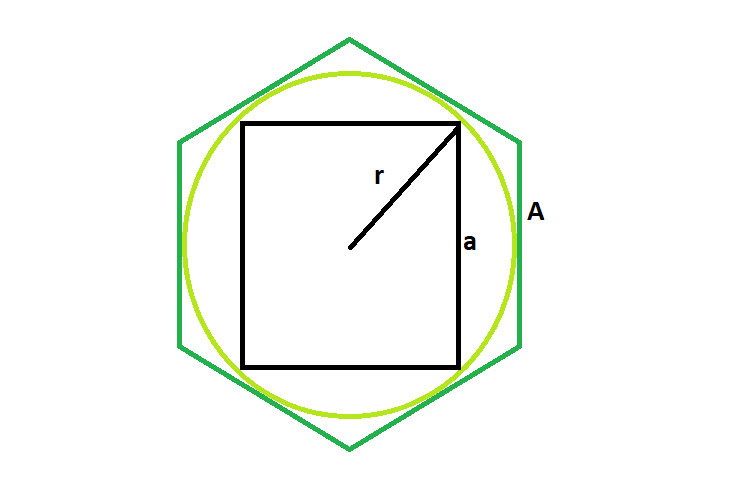# Area of a square inscribed in a circle which is inscribed in a hexagon

Given a regular hexagon with side A, which inscribes a circle of radius r, which in turn inscribes a square of side a.The task is to find the area of this square.

Examples:

```Input :  A = 5
Output : 37.5

Input : A = 8
Output : 96
```

## Recommended: Please try your approach on {IDE} first, before moving on to the solution.Approach:
We know the radius of the circle inscribed within the hexagon is, r=A√3/2(Please refer here)
Also, side length of circle within the circle is, a=√r=√3A/√2
So, Area of the Square, Area=(√3A/√2)^2

## C++

 `// C++ Program to find the area of the square ` `// inscribed within the circle which in turn ` `// is inscribed in a hexagon ` `#include ` `using` `namespace` `std; ` ` `  `// Function to find the area of the square ` `float` `area(``float` `a) ` `{ ` ` `  `    ``// side of hexagon cannot be negative ` `    ``if` `(a < 0) ` `        ``return` `-1; ` ` `  `    ``// area of the square ` `    ``float` `area = ``pow``((a * ``sqrt``(3)) / (``sqrt``(2)), 2); ` `    ``return` `area; ` `} ` ` `  `// Driver code ` `int` `main() ` `{ ` `    ``float` `a = 5; ` `    ``cout << area(a) << endl; ` `    ``return` `0; ` `} `

## Java

 `// Java Program to find the area of the square ` `// inscribed within the circle which in turn ` `// is inscribed in a hexagon ` ` `  `import` `java.io.*; ` ` `  `class` `GFG { ` ` `  `// Function to find the area of the square ` `static` `float` `area(``float` `a) ` `{ ` ` `  `    ``// side of hexagon cannot be negative ` `    ``if` `(a < ``0``) ` `        ``return` `-``1``; ` ` `  `    ``// area of the square ` `    ``float` `area = (``float``)Math.pow((a * Math.sqrt(``3``)) / (Math.sqrt(``2``)), ``2``); ` `    ``return` `area; ` `} ` ` `  `// Driver code ` `    ``public` `static` `void` `main (String[] args) { ` `        ``float` `a = ``5``; ` `    ``System.out.println( area(a)); ` `    ``} ` `} ` `// This code is contributed by ajit `

## Python3

 `# Python 3 Program to find the area  ` `# of the square inscribed within the  ` `# circle which in turn is inscribed  ` `# in a hexagon ` `from` `math ``import` `pow``, sqrt ` ` `  `# Function to find the area  ` `# of the square ` `def` `area(a): ` `     `  `    ``# side of hexagon cannot  ` `    ``# be negative ` `    ``if` `(a < ``0``): ` `        ``return` `-``1` ` `  `    ``# area of the square ` `    ``area ``=` `pow``((a ``*` `sqrt(``3``)) ``/`  `                   ``(sqrt(``2``)), ``2``) ` `    ``return` `area ` ` `  `# Driver code ` `if` `__name__ ``=``=` `'__main__'``: ` `    ``a ``=` `5` `    ``print``(``"{0:.3}"``.``format``(area(a))) ` ` `  `# This code is contributed  ` `# by SURENDRA_GANGWAR `

## C#

 `// C# Program to find the area of  ` `// the square inscribed within the  ` `// circle which in turn is inscribed  ` `// in a hexagon ` `using` `System; ` ` `  `class` `GFG ` `{ ` ` `  `// Function to find the area  ` `// of the square ` `static` `float` `area(``float` `a) ` `{ ` ` `  `    ``// side of hexagon cannot be negative ` `    ``if` `(a < 0) ` `        ``return` `-1; ` ` `  `    ``// area of the square ` `    ``float` `area = (``float``)Math.Pow((a * Math.Sqrt(3)) /  ` `                                 ``(Math.Sqrt(2)), 2); ` `    ``return` `area; ` `} ` ` `  `// Driver code ` `public` `static` `void` `Main () ` `{ ` `    ``float` `a = 5; ` `    ``Console.WriteLine( area(a)); ` `} ` `} ` ` `  `// This code is contributed by inder_verma.. `

## PHP

 ` `

Output:

```37.5
```

My Personal Notes arrow_drop_upCheck out this Author's contributed articles.

If you like GeeksforGeeks and would like to contribute, you can also write an article using contribute.geeksforgeeks.org or mail your article to contribute@geeksforgeeks.org. See your article appearing on the GeeksforGeeks main page and help other Geeks.

Please Improve this article if you find anything incorrect by clicking on the "Improve Article" button below.